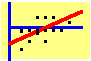8.6 APT Portfolio Calculation

APT provides a natural extension of the single-index model to accommodate a multi-factor return-generating process. In the single-index model, returns are explained by one factor, while the APT, and any multi-index model, assumes that returns are explained by K factors. This is useful when, for example, we believe that returns are affected not only by general market movements but also by factors such as the yield curve and inflationary expectations.

Recall that the return-generating process is: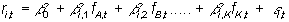Given this assumption, the variance of a portfolio's return is given by: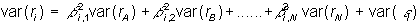That is, the variance equals the sum of the variances arising from the factors plus the unsystematic or idiosyncratic risk. Thus the objective function for the portfolio variance-minimization problem reduces to: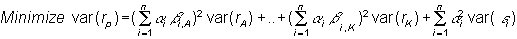By comparing this equation for portfolio variance with the single-index model: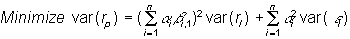you can see that each additional factor increases the computational complexity of the model.

However, the basic principles remain the same. The optimal portfolio weights are solved, given some target return, to minimize portfolio variance.

previous topic